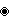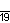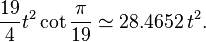Kids Encyclopedia Facts
Quick facts for kidsType Regular polygon
Edges and vertices 19
Schläfli symbol {19}
Coxeter diagramSymmetry group Dihedral (D19), order 2×19
Internal angle (degrees) ≈161.052°
Dual polygon Self
Properties Convex, cyclic, equilateral, isogonal, isotoxal

A enneadecagon or 19-gon is a shape with 19 sides and 19 corners. It is also known as an enneakaidecagon or a nonadecagon.

The radius of the circumcircle of the regular enneadecagon with side length t is$R=\frac{t}{2} \csc \frac {180}{19}$ (angle in degrees). The area, where t is the edge length, is$\frac{19}{4}t^2 \cot \frac{\pi}{19} \simeq 28.4652\,t^2.$